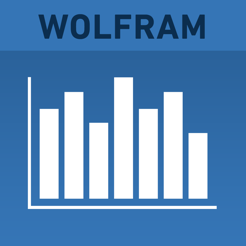## Screenshots

•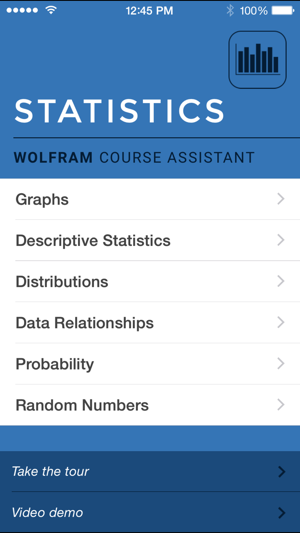•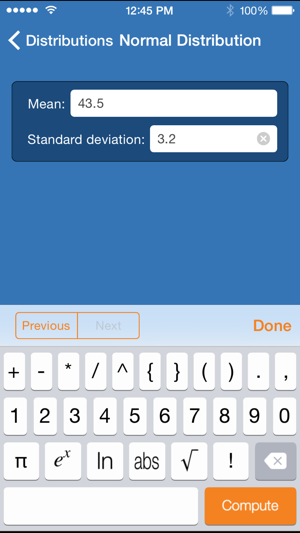•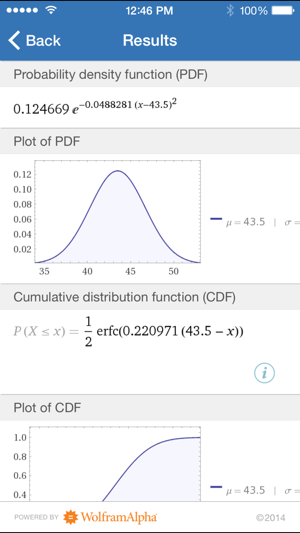•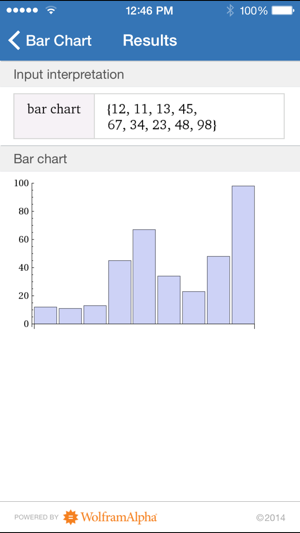•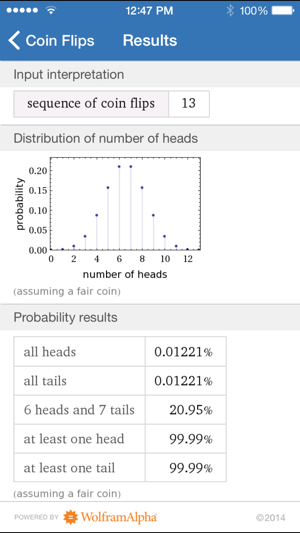## Description

Taking statistics? Then you need the Wolfram Statistics Course Assistant. This definitive app for statistics—from the world leader in math software—will help you work through your homework problems, ace your tests, and learn statistics concepts. Forget canned examples! The Wolfram Statistics Course Assistant solves your specific problems on the fly, covering descriptive statistics, distributions, and much more.

This app covers the following topics applicable to Statistics and Introduction to Statistics:

- Create a bar chart, histogram, or scatter plot of any set of data
- Find the mean, median, mode, standard deviation, quartiles, and interquartile range of a dataset
- Calculate normal probabilities and find information about the normal distribution
- Calculate binomial probabilities and find information about the binomial distribution
- Compute probabilities based on dice rolls and coin flips
- Find the best-fit line of a set of data points
- Select random integers or random real numbers

The Wolfram Statistics Course Assistant is powered by the Wolfram|Alpha computational knowledge engine and is created by Wolfram Research, makers of Mathematica—the world's leading software system for mathematical research and education.

The Wolfram Statistics Course Assistant draws on the computational power of Wolfram|Alpha's supercomputers over a 3G, 4G, or Wi-Fi connection.

Version 1.3.1

- Bug fixes

## Ratings and Reviews

1.3 out of 5
3 Ratings

3 Ratings

Katherine_LI_NY ,

### Terrible app

This app is a ***terrible*** tool for a statistics class. The interface is not user friendly and it is painfully difficult to input data. There is also no way to compare across data sets once each set is entered - you have to start all over again. Most of the tools such as those for probability and data relationships, will never be of use to a real student in a class...the tools that we actually need, like for ANOVA, are not included.

Gauss743 ,

### Useful but limited

This app computes several basic statistics for selected distributions such as the normal and binomial. Basic descriptive statistics are also computed for arbitrary data sets input by the user. Bar charts, histograms, and scatterplots are produced and displayed.
The app's best feature is the calculation of probabilities for several distributions.
The limitations are serious but not disabling. Graphing and descriptive statistics are limited by the fact that data entry is strictly manual, one data point at a time. This means that only small data sets are practical to use.
The worst limitation is that there is no means to save or print the results. So a student using the app for homework must copy the output by hand. This is not an issue for numerical output such as a mean or a probability, but it is a big issue for the plots.
The best feature of the app and the main reason I would recommend the app to my statistics students is the probability calculations, which allow the student to avoid using tables.

rob66569 ,

### Data Science

Statistics is about data, and the biggest drawback of this app is that the interface for inputting data is lousy. At best, this serves as a simple calculator for toy problems. No way any student could examine a real data set with hundreds of rows and more than two variables. Even including two variables is awkward here, because they must be input in (x1,y1), (x2,y2)....format.

The graphics are fine (hence two stars) and there are some nice interactive touches, but this is a toy. As an intermediate step between toy and data analysis device, it might be nice if they would include some pre-installed real data sets that include an interesting context and demonstrate that the analyses actually can contribute to knowledge about real phenomenon.

## Information

Seller
Wolfram Group LLC
Size
13.2 MB
Category
Education
Compatibility

Requires iOS 9.3 or later. Compatible with iPhone, iPad, and iPod touch.

Languages

English

Age Rating
4+
Price
\$1.99

## Supports

•